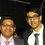# Clarification in a problem

Hey Guys, I have this question but my friend and I are getting different answers, and I don't have the answer booklet with me. Could you guys help ?

So, here's the question.

Find the number of all possible k-tuples of non - negative integers (n(1),n(2),n(3).... n(k)) such that

sum_{i=1}^k n(i) = 100

So, this is the question. Now here's my solution.

Let there be 100 stars placed in a line. We have to divide these 100 stars into k groups such that no group equals 0 . So, let me do the division between stars by placing a bar between the stars. By this way, there are 99 possible ways in which I can set a bar (between any two stars) . But as I only have to create k groups, I need only place k-1 bars..

So I have 99 places and I need to choose any (k-1) places.. SO, I get the answer as

99 C (k-1)

Is it correct guys ? Because I have a friend who says the answer is (99+k)C(k-1).Note by Adeetya Tantia
7 years, 1 month ago

This discussion board is a place to discuss our Daily Challenges and the math and science related to those challenges. Explanations are more than just a solution — they should explain the steps and thinking strategies that you used to obtain the solution. Comments should further the discussion of math and science.

When posting on Brilliant:

• Use the emojis to react to an explanation, whether you're congratulating a job well done , or just really confused .
• Ask specific questions about the challenge or the steps in somebody's explanation. Well-posed questions can add a lot to the discussion, but posting "I don't understand!" doesn't help anyone.
• Try to contribute something new to the discussion, whether it is an extension, generalization or other idea related to the challenge.
• Stay on topic — we're all here to learn more about math and science, not to hear about your favorite get-rich-quick scheme or current world events.

MarkdownAppears as
*italics* or _italics_ italics
**bold** or __bold__ bold
- bulleted- list
• bulleted
• list
1. numbered2. list
1. numbered
2. list
Note: you must add a full line of space before and after lists for them to show up correctly
paragraph 1paragraph 2

paragraph 1

paragraph 2

[example link](https://brilliant.org)example link
> This is a quote
This is a quote
    # I indented these lines
# 4 spaces, and now they show
# up as a code block.

print "hello world"
# I indented these lines
# 4 spaces, and now they show
# up as a code block.

print "hello world"
MathAppears as
Remember to wrap math in $$ ... $$ or $ ... $ to ensure proper formatting.
2 \times 3 $2 \times 3$
2^{34} $2^{34}$
a_{i-1} $a_{i-1}$
\frac{2}{3} $\frac{2}{3}$
\sqrt{2} $\sqrt{2}$
\sum_{i=1}^3 $\sum_{i=1}^3$
\sin \theta $\sin \theta$
\boxed{123} $\boxed{123}$

## Comments

Sort by:

Top Newest

Your friend is correct.

If the problem asked about positive integers, then your answer of $\dbinom{99}{k - 1}$ would be correct. But the $n_i$ are nonnegative, which means they can be 0. Your approach does not account for this.

Instead, consider any arrangement of 100 stars and $k - 1$ bars. (In particular, there could be two or more bars between consecutive stars.) Then you take $n_1$ as the number of stars before the first bar, $n_2$ as the number of stars between the first bar and second bar, and so on. This gives you solutions where the $n_i$ can be 0. Hence, the number of solutions is the number of ways of arranging 100 stars and $k - 1$ bars, which is $\binom{100 + (k - 1)}{100} = \binom{k + 99}{100} = \binom{k + 99}{k - 1}.$

- 7 years, 1 month ago

Log in to reply

Thanks.

- 7 years, 1 month ago

Log in to reply

Use the Stars and Bars formula, it's the same thing. A standard result !

- 6 years, 11 months ago

Log in to reply

×

Problem Loading...

Note Loading...

Set Loading...